Technical Article

June 21, 2016 by Robert Keim

## In this article, we’ll discuss two benefits of using an active load: improved biasing conditions and differential-to-single-ended conversion.

In this article, we’ll discuss two benefits of using an active load: improved biasing conditions and differential-to-single-ended conversion.

### Better Biasing

The previous article presented the “drain-resistor problem”: We need a larger drain resistor to achieve higher gain, but more drain resistance means a lower DC bias voltage at the output node.

This is a problem because the output voltage is also the MOSFET’s drain voltage, and a lower drain voltage corresponds to a higher risk of pushing the FET out of saturation and into the triode region. We suggested that a current source might resolve this problem by providing high gain without negatively affecting the bias conditions.

The following diagram gives you an idea of the improved biasing situation associated with the use of a current mirror instead of drain resistors.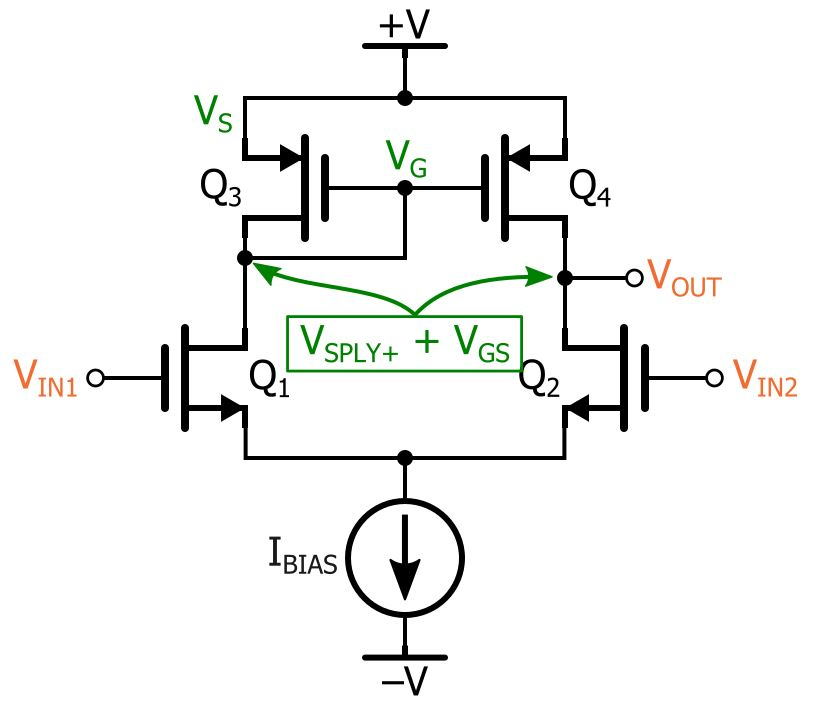We have not yet discussed the small-signal resistance of the active-load current mirror, so for now you’ll just have to believe me when I tell you that it is quite high. But as you can see in the circuit diagram, this large small-signal resistance does not apply to the biasing conditions: The bias voltage at the output node is determined by whatever gate-to-source voltage corresponds to Q3’s drain current.

If we consider that this drain current is not particularly large and that Q3’s threshold voltage is maybe 0.7 V, we can guess that the magnitude of VGS will be quite small relative to the high gain resulting from the current mirror’s large small-signal resistance.

Let’s confirm this guess via simulation. Here is the LTspice circuit, with the bias voltage labeled: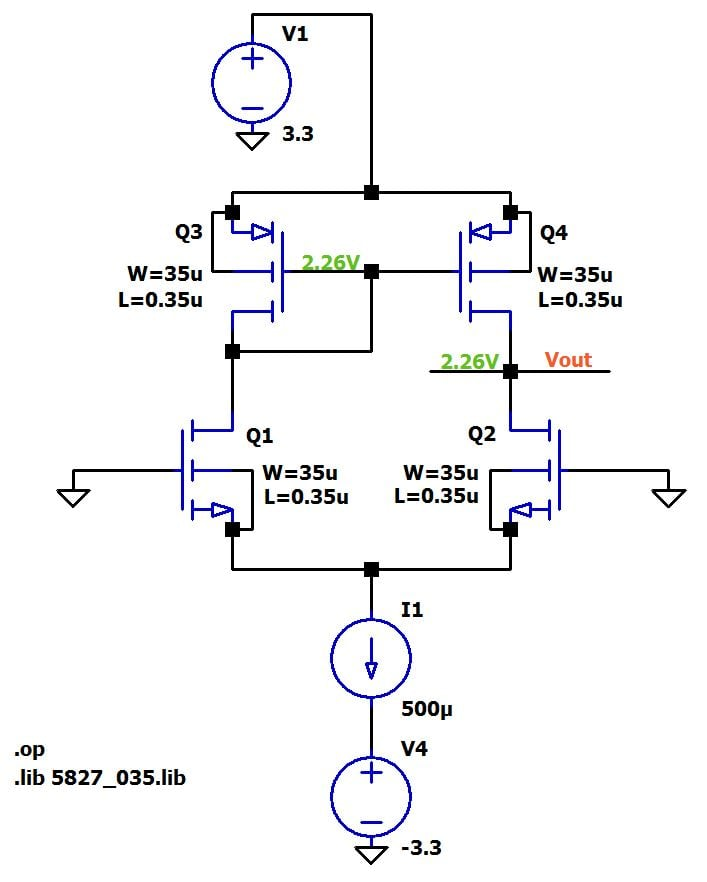(The SPICE models for the FETs can be downloaded here.) This simulation demonstrates that the current mirror’s large small-signal resistance (and thus high gain) does not require a large DC voltage drop. With this particular circuit, Q3 can generate current equal to IBIAS/2 (i.e., 250 µA) with a gate-to-source voltage of only –1.04 V, leading to an output-node bias voltage of (3.3 + VGS) = 2.26 V.

While we’re on this subject, I should point out that the bias voltage will be influenced by the width-to-length ratio of the current-mirror transistors. Recall that the saturation-mode relationship between gate-to-source voltage and drain current (if we ignore channel-length modulation) is the following:

$I_D=\frac{1}{2}\mu_nC_{ox}\frac{W}{L}(V_{GS}-V_{TH})^2$

We can see that a lower width-to-length ratio will cause the FET to conduct less drain current for the same VGS. Likewise, if the drain current is held constant and the width-to-length ratio is reduced, the magnitude of VGS will have to increase. Theoretically, then, we could fine-tune the bias voltage by adjusting the width-to-length ratio of the current-mirror transistors. Consider the following simulation: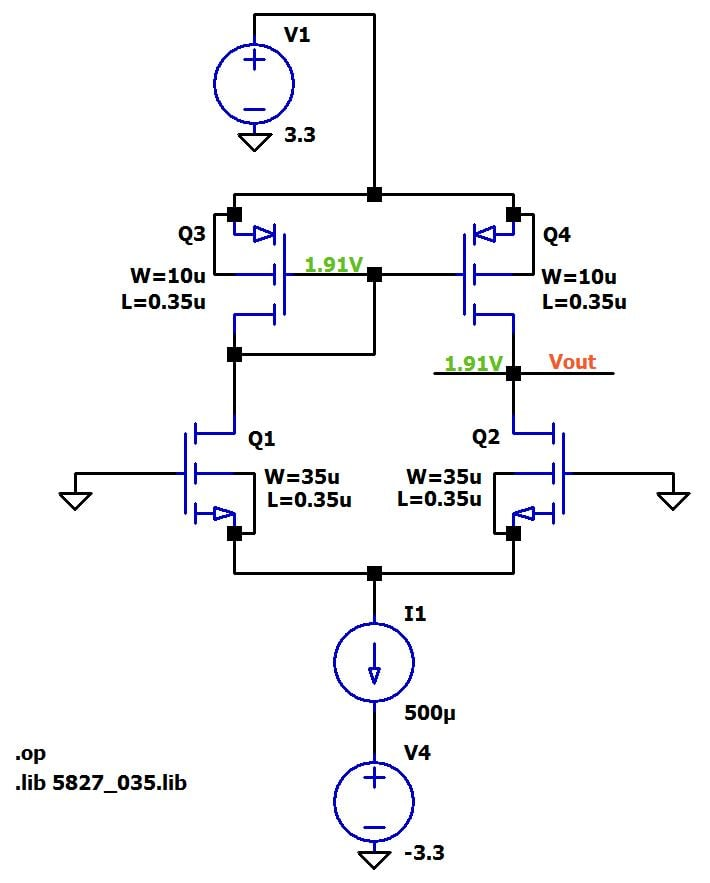The output-voltage range is restricted by the positive supply voltage and the lowest voltage that allows Q2 to remain in saturation. The condition for saturation is VGD ≤ VTH, so this lower limit is equal to VTH volts below the DC offset of the voltage applied to the gate of Q2. In this circuit we have a DC offset of 0 V and VTH for the NMOS transistors is ~0.5 V, so the lower limit is around –0.5 V. Thus, by reducing the width to 10 µm, we have moved the output node’s bias voltage closer to the middle of the allowable range.

Before we move on, I should point out that in real life the biasing scheme shown above is not practical. The VOUT bias voltage is very important: If it’s too high (or too low), the output’s positive (or negative) signal swing will be restricted. The biasing accomplished in our simulations is reliable only because Q3 and Q4 are perfectly matched.

We can rely on IC manufacturing technology to achieve good matching, but not perfect matching, and any mismatches between the two transistors will lead to variations in the VOUT bias voltage. Thus, real-life implementations employ special biasing circuits that are not so sensitive to manufacturing imperfections.

### Two Outputs or One?

You may have noticed that the left-hand VOUT disappeared when we switched from drain resistors to a current mirror. It turns out that an additional (and perhaps somewhat unexpected) benefit of active loading is that it converts the output signal from differential to single-ended without loss of gain.

If you have read The Basic MOSFET Differential Pair, you might remember that we analyzed the overall gain differentially, meaning the output signal was defined as VOUT1 – VOUT2. These two signals are 180° out of phase, so the amplitude of the resulting output signal is doubled.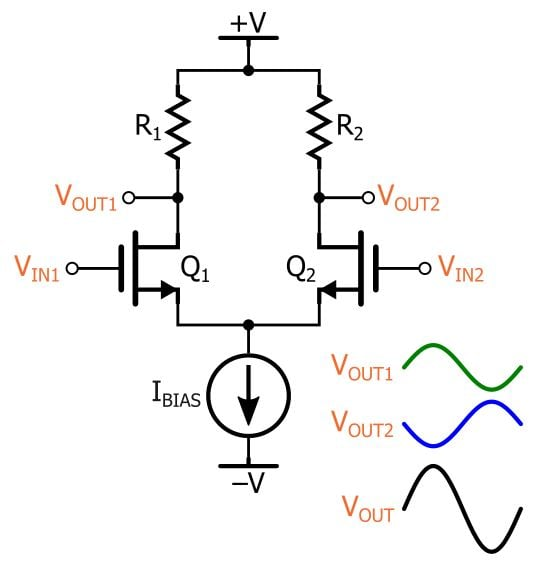In many cases, though, we don’t want differential output signals. The easiest way to make the output single-ended is to simply ignore either VOUT1 or VOUT2, but this will reduce our gain by a factor of 2. It is extremely convenient, then, that the active-load configuration performs the differential-to-single-ended conversion without reducing the gain. Let’s take a conceptual look at how it accomplishes this feat.

Keep in mind that this will be a small-signal analysis—we are concerned only with how the circuit responds to small changes in voltage or current. These small changes can be thought of as a separate “small signal” that is superimposed on a biasing signal. I will use lowercase letters to refer to these small signals (e.g., vgs, id). Don’t let this small-signal technique distract you from the fact that the small signals don’t really exist as separate entities; they simply represent small changes in the large-signal (i.e., DC bias) quantities. The reason we treat them as separate entities is because small-signal analysis can ignore the DC component of the signals and still produce accurate results.

When performing small-signal analysis, we replace constant DC current sources with open circuits and constant DC voltage sources with short circuits. Thus, we will use the following circuit diagram: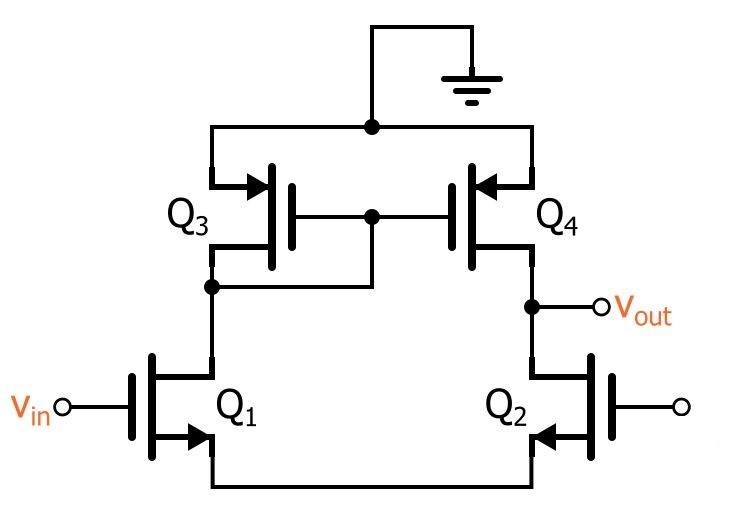So, if we apply a positive small-signal input vin, we will get a small-signal drain current id flowing downward through Q1. This would lead to an output voltage equal to the drain current multiplied by the drain resistance (whether this is an actual drain resistor or the small-signal resistance of Q3). So far there is no fundamental difference between the active-load configuration and the drain-resistor configuration.

Notice, however, that this drain current will continue flowing along the bottom of the circuit and up through Q2: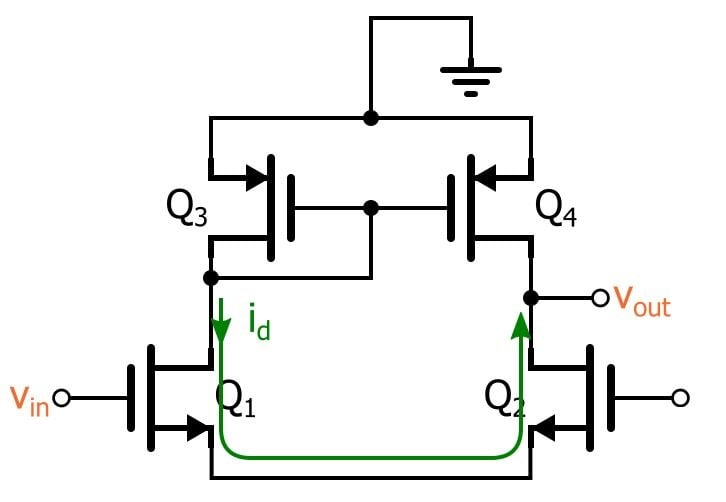So we have id flowing up toward the output node. But let’s not forget about the active-load current mirror—assuming that the two current-mirror FETs have the same width-to-length ratio, Q4 will duplicate Q3’s drain current, as follows: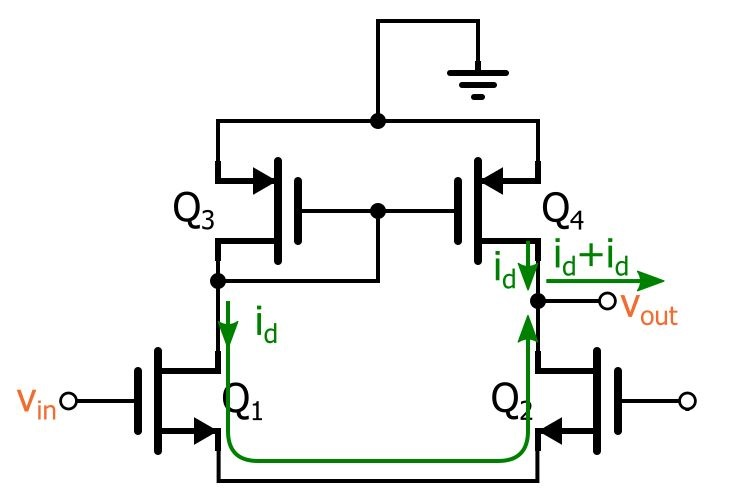Thus, we have two currents equal to id that meet at the output node. The resulting current magnitude is id + id = 2id, and this doubling of output current is why the active-load configuration achieves the full differential gain while utilizing only one output signal.

### Conclusion

I hope that you now understand the two active-load benefits—i.e., improved biasing and differential-to-single-ended conversion—covered in this article. However, our interest in these benefits is closely linked to the expectation that the active load can also give us higher gain compared to a drain-resistor implementation.

In the next article, then, we’ll analyze this circuit with respect to the differential gain.

Next Article in Series:• A
anhnha June 25, 2016

Thanks a lot for the great article. It is really interesting. I would like to read more about sub-circuits used in integrated circuits.

Like.
• A